Mathematics: CUET Mock Test - 3

# Mathematics: CUET Mock Test - 3

Test Description

## 40 Questions MCQ Test CUET Science Subjects Mock Tests | Mathematics: CUET Mock Test - 3

Mathematics: CUET Mock Test - 3 for Class 12 2023 is part of CUET Science Subjects Mock Tests preparation. The Mathematics: CUET Mock Test - 3 questions and answers have been prepared according to the Class 12 exam syllabus.The Mathematics: CUET Mock Test - 3 MCQs are made for Class 12 2023 Exam. Find important definitions, questions, notes, meanings, examples, exercises, MCQs and online tests for Mathematics: CUET Mock Test - 3 below.
Solutions of Mathematics: CUET Mock Test - 3 questions in English are available as part of our CUET Science Subjects Mock Tests for Class 12 & Mathematics: CUET Mock Test - 3 solutions in Hindi for CUET Science Subjects Mock Tests course. Download more important topics, notes, lectures and mock test series for Class 12 Exam by signing up for free. Attempt Mathematics: CUET Mock Test - 3 | 40 questions in 45 minutes | Mock test for Class 12 preparation | Free important questions MCQ to study CUET Science Subjects Mock Tests for Class 12 Exam | Download free PDF with solutions
 1 Crore+ students have signed up on EduRev. Have you?
Mathematics: CUET Mock Test - 3 - Question 1

### Bag 1 contains 4 white and 6 black balls while another Bag 2 contains 4 white and 3 black balls. One ball is drawn at random from one of the bags and it is found to be black. Find the probability that it was drawn from Bag 1.

Detailed Solution for Mathematics: CUET Mock Test - 3 - Question 1

Let E1 = event of choosing the bag 1, E2 = event of choosing the bag 2.
Let A be event of drawing a black ball.
P(E1) = P(E2) = 1/2.
Also, P(A|E1) = P(drawing a black ball from Bag 1) = 6/10 = 3/5.
P(A|E2) = P(drawing a black ball from Bag 2) = 3/7.
By using Bayes’ theorem, the probability of drawing a black ball from bag 1 out of two bags is-:
P(E1 | A) = P(E1)P(A | E1)/( P(E1)P(A│E1)+P(E2)P(A | E2))
= (1/2 × 3/5) / ((1/2 × 3/7)) + (1/2 × 3/5)) = 7/12.

Mathematics: CUET Mock Test - 3 - Question 2

### Formula for Bayes theorem is ________

Detailed Solution for Mathematics: CUET Mock Test - 3 - Question 2

Bayes theorem formula is P(A | B) =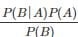The formula provides relationship between P(A | B) and P(B | A). It is mainly derived from conditional probability formula P(A | B) and P(B | A). Where,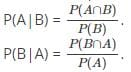Mathematics: CUET Mock Test - 3 - Question 3

### Previous probabilities in Bayes Theorem that are changed with the new available information are called _____

Detailed Solution for Mathematics: CUET Mock Test - 3 - Question 3

In Bayesian statistics, we calculate new probability after information becomes available due to new events and this is known as Posterior Probability. There is no term like Independent probabilities and Dependent probabilities, there are only independent events and dependent events. Interior probabilities represent probabilities of the intersection between two events.

Mathematics: CUET Mock Test - 3 - Question 4

Bag 1 contains 3 red and 5 black balls while another Bag 2 contains 4 red and 6 black balls. One ball is drawn at random from one of the bags and it is found to be red. Find the probability that it is drawn from bag 2.

Detailed Solution for Mathematics: CUET Mock Test - 3 - Question 4

Let E1 = event of choosing the bag 1, E2 = event of choosing the bag 2.
Let A be event of drawing a red ball.
P(E1) = P(E2) = 1/2.
Also, P(A | E1) = P(drawing a red ball from Bag 1) = 3/8.
And P(A | E2) = P(drawing a red ball from Bag 2) = 4/10.
The probability of drawing a ball from bag 2, being given that it is red is P(E2 | A).
By using Bayes’ theorem,
P(E2 | A) = P(E2)P(A | E2)/( P(E1)P(A│E1)+P(E2)P(A | E2))
= (1/2 × 4/10) / ((1/2 × 3/8)) + (1/2 × 4/10)) = 16/31.

Mathematics: CUET Mock Test - 3 - Question 5

_____ is the complement of the angle between the line L and a normal line to the plane π.

Detailed Solution for Mathematics: CUET Mock Test - 3 - Question 5

The angle between a line and a plane is the complement of the angle between the line L and a normal line to the plane π. If θ is the angle between line whose ratios are a1, b1, c1 and the plane ax + by + cz + d = 0 then sin θ =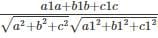.

Mathematics: CUET Mock Test - 3 - Question 6

Find the angle between the planes x + 2y + 3z + 1 = 0 and (4, 1, -7).

Detailed Solution for Mathematics: CUET Mock Test - 3 - Question 6

Angle between a plane and a line sin θ =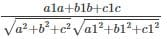sin θ = – 0.49
θ = sin-1(- 0.49)
θ = – 29.34

Mathematics: CUET Mock Test - 3 - Question 7

What is the plane equation involved in the formula sinθ =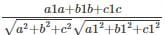?

Detailed Solution for Mathematics: CUET Mock Test - 3 - Question 7

The angle between a line and a plane is the complement of the angle between the line L and a normal line to the plane π. If θ is the angle between line whose ratios are a1, b1, c1 and the plane ax + by + cz + d = 0 then sin θ =Mathematics: CUET Mock Test - 3 - Question 8

What is the relation between the plane ax + by + cz + d = 0 and a1, b1, c1 the direction ratios of a line, if the plane and line are parallel to each other?

Detailed Solution for Mathematics: CUET Mock Test - 3 - Question 8

The relation between the plane ax + by +cz + d = 0 and a1, b1, c1 the direction ratios of a line, if the plane and line are parallel to each other is a1a + b1b + c1c = 0.

Mathematics: CUET Mock Test - 3 - Question 9

The condition a1a + b1b + c1c = 0 is for a plane and a line are _____ to each other.

Detailed Solution for Mathematics: CUET Mock Test - 3 - Question 9

The relation between the plane ax + by +cz + d = 0 and a1, b1, c1 the direction ratios of a line, if the plane and line are parallel to each other is a1a + b1b + c1c = 0.

Mathematics: CUET Mock Test - 3 - Question 10

Find the angle between 2x + 3y – 2z + 4 = 0 and (2, 1, 1).

Detailed Solution for Mathematics: CUET Mock Test - 3 - Question 10

Angle between a plane and a line sin θ =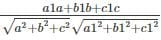sinθ = 0.49
θ = sin-1(0.49)
θ = 29.34

Mathematics: CUET Mock Test - 3 - Question 11

The plane 5x + y + kz + 1 = 0 and directional ratios of a line (3, -1, 1) are parallel, find k.

Detailed Solution for Mathematics: CUET Mock Test - 3 - Question 11

The condition for a plane and a line are parallel to each other is a1a + b1b + c1c = 0.
5(3) + 1(-1) + k(1) = 0
K(1) = -14
K = -14

Mathematics: CUET Mock Test - 3 - Question 12

Find k for the given plane x + 2y + kz + 2 = 0 and directional ratios of a line (8, 3, 2), if they are parallel to each other.

Detailed Solution for Mathematics: CUET Mock Test - 3 - Question 12

The condition for a plane and a line are parallel to each other is a1a + b1b + c1c = 0.
8(1) + 3(2) + 2(k) = 0
2(k) = -14
k = -7

Mathematics: CUET Mock Test - 3 - Question 13

If θ is the angle between line whose ratios are a1, b1, c1 and the plane ax + by + cz + d = 0 then
cos θ =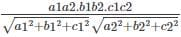Detailed Solution for Mathematics: CUET Mock Test - 3 - Question 13

A mathematical symbol θ is used to find the angle between line and a normal line to the plane π along with a trigonometric function called sine. Hence, the formula
sin θ =Mathematics: CUET Mock Test - 3 - Question 14

Which trigonometric function is used to find the angle between a line and a plane?

Detailed Solution for Mathematics: CUET Mock Test - 3 - Question 14

The trigonometric function is used to find the angle between a line and a plane is sine. If θ is the angle between line whose ratios are a1, b1, c1 and the plane ax + by + cz + d = 0 then sin θ =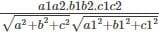.

Mathematics: CUET Mock Test - 3 - Question 15

A plane and a line having an angle of 90 degrees between them are called _____

Detailed Solution for Mathematics: CUET Mock Test - 3 - Question 15

A plane and A line which are perpendicular to each other or a plane and a line having an angle 90 degrees between them are called orthogonal. θ is equal to 90 degrees in sin θ =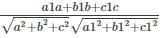.

Mathematics: CUET Mock Test - 3 - Question 16

The condition a/a1 = b/b1 = c/c1 is for a plane and a line are _____ to each other.

Detailed Solution for Mathematics: CUET Mock Test - 3 - Question 16

θ = 90 degrees
The relation between the plane ax + by + cz + d = 0 and a1, b1, c1 the direction ratios of a line, if the plane and line are perpendicular to each other is a/a, = b/b1 = c/c1.

Mathematics: CUET Mock Test - 3 - Question 17

Find the angle between x + 2y + 7z + 2 = 0 and (2, 4, 6).

Detailed Solution for Mathematics: CUET Mock Test - 3 - Question 17

Angle between a plane and a line sin θ =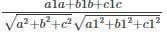.
sinθ = 0.92
θ = 66.92

Mathematics: CUET Mock Test - 3 - Question 18

Find the angle between the planes 5x + 2y + 3z + 1 = 0 and (1, 1, -2).

Detailed Solution for Mathematics: CUET Mock Test - 3 - Question 18

Angle between a plane and a line sin θ =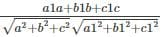.
sinθ = 0.06
θ = 3.43

Mathematics: CUET Mock Test - 3 - Question 19

_____ is the angle between the normals to two planes.

Detailed Solution for Mathematics: CUET Mock Test - 3 - Question 19

The angle between the normals to two planes is called the angle between the planes. A trigonometric identity, cosine is used to find the angle called ‘θ’ between two planes.

Mathematics: CUET Mock Test - 3 - Question 20

What is the formula to find the angle between the planes a1x + b1y + c1z + d1 = 0 and a2x + b2y + c2z + d2 = 0?

Detailed Solution for Mathematics: CUET Mock Test - 3 - Question 20

The formula to find the angle between the planes a1x + b1y + c1z + d1 = 0 and a2x + b2y + c2z + d2 = 0 is cos θ =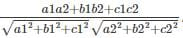.
θ is the angle between the normal of two planes.

Mathematics: CUET Mock Test - 3 - Question 21

Find s for the given planes 2x + 2y + sz + 2 = 0 and 3x + y + z – 2 = 0, if they are perpendicular to each other.

Detailed Solution for Mathematics: CUET Mock Test - 3 - Question 21

If their normals are perpendicular to each other then a1a2 + b1b2 + c1c2 = 0.
2(3) + 2(1) + s(1) = 0
s(1) = - 8
k = - 8

Mathematics: CUET Mock Test - 3 - Question 22

What is the relation between the planes a1x + b1y + c1z + d1 = 0 and a2x + b2y + c21z + d2 = 0, if their normal are perpendicular to each other?

Detailed Solution for Mathematics: CUET Mock Test - 3 - Question 22

θ = 90 degrees ⇒ cos θ
a1a2 + b1b2 – c1c2 = 0
Relation between the planes a1x + b1y + c1z + d1 = 0 and a2x + b2y + c21z + d2 = 0, if their normal are perpendicular to each other is a1a2 + b1b2 + c1c2 = 0.

Mathematics: CUET Mock Test - 3 - Question 23

The planes 5x + y + 3z + 1 = 0 and x + y – kz + 6 = 0 are orthogonal, find k.

Detailed Solution for Mathematics: CUET Mock Test - 3 - Question 23

Relation between the planes a1x + b1y + c1z + d1 = 0 and a2x + b2y + c21z + d2 = 0, if their normal are perpendicular to each other is a1a2 + b1b2 + c1c2 = 0.
5(1) + 1(1) + 3(-k) = 0
-3k = -6
K = 2

Mathematics: CUET Mock Test - 3 - Question 24

Find k for the given planes x + 2y + kz + 2 = 0 and 3x + 4y – z + 2 = 0, if they are perpendicular to each other.

Detailed Solution for Mathematics: CUET Mock Test - 3 - Question 24

Relation between the the planes a1x + b1y + c1z + d1 = 0 and a2x + b2y + c21z + d2 = 0, if their normal are perpendicular to each other is a1a2 + b1b2 + c1c2 = 0.
1(3) + 2(4) + k(-1) = 0
k(-1) = -11
k = 11

Mathematics: CUET Mock Test - 3 - Question 25

Which of the following is not the correct formula for representing a plane?

Detailed Solution for Mathematics: CUET Mock Test - 3 - Question 25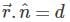, ax + by + cz = d, lx + my + nz = d are the various ways of representing a plane.is the vector equation of the plane, where n^ is the unit vector normal to the plane.
ax + by + cz = d, lx + my + nz = d are the Cartesian equation of the plane in the normal form where, a, b, c are the direction ratios and l, m, n are the direction cosines of the normal to the plane respectively.

Mathematics: CUET Mock Test - 3 - Question 26

Find the vector equation of the plane which is at a distance of 7/√38 from the origin and the normal vector from origin is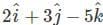?

Detailed Solution for Mathematics: CUET Mock Test - 3 - Question 26

Let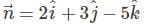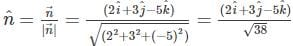Hence, the required equation of the plane is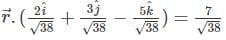Mathematics: CUET Mock Test - 3 - Question 27

Find the Cartesian equation of the plane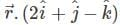= 4.

Detailed Solution for Mathematics: CUET Mock Test - 3 - Question 27

Given that the equation of the plane is= 4.
We know that,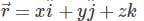∴⇒ 2x + y - z = 4 is the Cartesian equation of the plane.

Mathematics: CUET Mock Test - 3 - Question 28

Find the distance of the plane 3x + 4y - 5z - 7=0.

Detailed Solution for Mathematics: CUET Mock Test - 3 - Question 28

From the given equation, the direction ratios of the normal to the plane are 3, 4, -5; the direction cosines are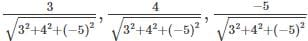, i.e. 3√50,4√50,−5√50
Dividing the equation throughout by √50, we get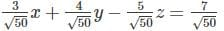The above equation is in the form of lx + my + nz = d, where d is the distance of the plane from the origin. So, the distance of the plane from the origin is 7√50.

Mathematics: CUET Mock Test - 3 - Question 29

Find the equation of the plane passing through the three points (2,2,0), (1,2,1), (-1,2,-2).

Detailed Solution for Mathematics: CUET Mock Test - 3 - Question 29

Let,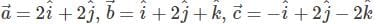The vector equation of the plane passing through three points is given by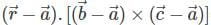= 0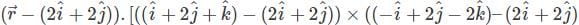= 0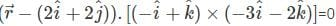Mathematics: CUET Mock Test - 3 - Question 30

Find the Cartesian equation of the plane passing through the point (3,2,-3) and the normal to the plane is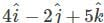?

Detailed Solution for Mathematics: CUET Mock Test - 3 - Question 30

The position vector of the point (3,2,-3) is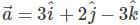and the normal vector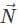perpendicular to the plane is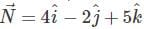Therefore, the vector equation of the plane is given by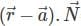= 0
Hence,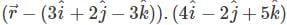= 0
4(x - 3)-2(y-2) + 5(z + 3) = 0
4x - 2y + 5z + 7=0.

Mathematics: CUET Mock Test - 3 - Question 31

Find the Cartesian equation of the plane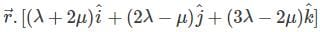= 12

Detailed Solution for Mathematics: CUET Mock Test - 3 - Question 31

Given that the equation of the plane isWe know that,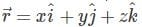∴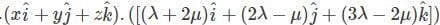= 12
⇒ (λ + 2μ)x + (2λ - μ)y + (3λ - 2μ)z = 12 is the Cartesian equation of the plane.

Mathematics: CUET Mock Test - 3 - Question 32

Find the Cartesian equation of the plane passing through the point (3,2,-3) and the normal to the plane is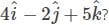Detailed Solution for Mathematics: CUET Mock Test - 3 - Question 32

The position vector of the point (3,2,-3) is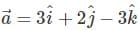and the normal vector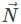perpendicular to the plane is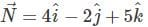Therefore, the vector equation of the plane is given by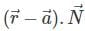= 0
Hence,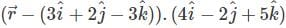= 0
4(x - 3) - 2(y - 2) + 5(z + 3) = 0
4x - 2y + 5z + 7 = 0

Mathematics: CUET Mock Test - 3 - Question 33

Evaluate the product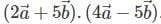Detailed Solution for Mathematics: CUET Mock Test - 3 - Question 33

To evaluate: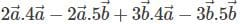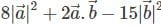Mathematics: CUET Mock Test - 3 - Question 34

Find the vector product of the vectors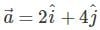and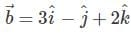.

Detailed Solution for Mathematics: CUET Mock Test - 3 - Question 34

Explanation: Given that,andCalculating the vector product, we get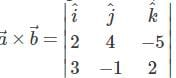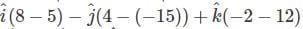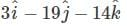Mathematics: CUET Mock Test - 3 - Question 35

Find the vector product of the vectors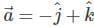and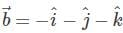.

Detailed Solution for Mathematics: CUET Mock Test - 3 - Question 35

Given that,andCalculating the vector product, we get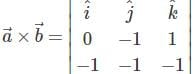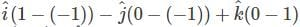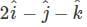Mathematics: CUET Mock Test - 3 - Question 36

Find the magnitude of a⃗  and b⃗  which are having the same magnitude and such that the angle between them is 60° and their scalar product is 1/4.

Detailed Solution for Mathematics: CUET Mock Test - 3 - Question 36

Given that: a)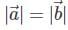b) θ = 60° and
c)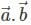= 14
∴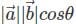= 1/4
= |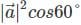= 1/4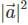= 1/4 x 1/2
∴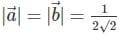Mathematics: CUET Mock Test - 3 - Question 37

If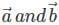are two non-zero vectors then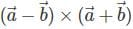= _________

Detailed Solution for Mathematics: CUET Mock Test - 3 - Question 37

Consider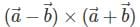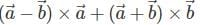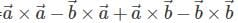We know that,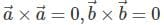and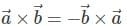∴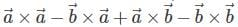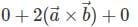Hence,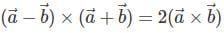Mathematics: CUET Mock Test - 3 - Question 38

Direction of λ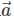andis same if λ is _______

Detailed Solution for Mathematics: CUET Mock Test - 3 - Question 38

Direction of λandis same if value λ is positive as it gives it a direction which is positive in nature. If the value of λ is negative then the direction of the result after multiplication becomes in opposite direction. Whereas the value of the product vector becomes zero if value of λ is 0.

Mathematics: CUET Mock Test - 3 - Question 39

|λ| times the magnitude of vector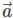is denoted as ______

Detailed Solution for Mathematics: CUET Mock Test - 3 - Question 39

|λ| times the magnitude of vectoris denoted as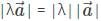As we know that the magnitude of vectoris denoted by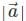, if we multiply the magnitude of vectorwith magnitude of λ we get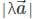.

Mathematics: CUET Mock Test - 3 - Question 40

Find values of x, y, z if vectors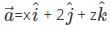and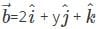are equal.

Detailed Solution for Mathematics: CUET Mock Test - 3 - Question 40

As both the vectors are equal hence, we can equate their constants and get the value of x, y and z. Now we equate the coefficients of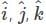of both the equations and get the values
x = 2, y = 2, z = 1.

## CUET Science Subjects Mock Tests

60 tests
 Use Code STAYHOME200 and get INR 200 additional OFF Use Coupon Code
Information about Mathematics: CUET Mock Test - 3 Page
In this test you can find the Exam questions for Mathematics: CUET Mock Test - 3 solved & explained in the simplest way possible. Besides giving Questions and answers for Mathematics: CUET Mock Test - 3, EduRev gives you an ample number of Online tests for practice

60 tests